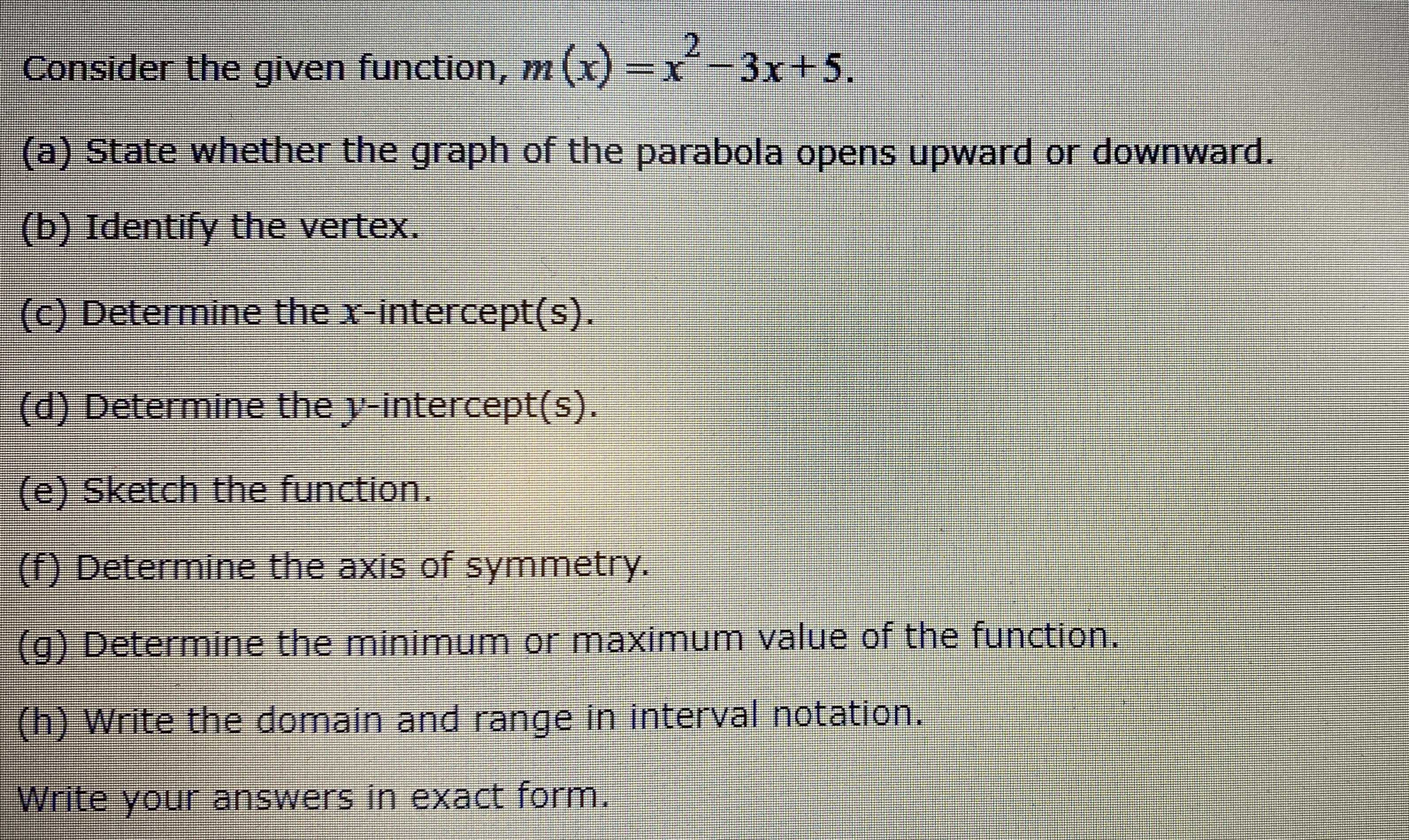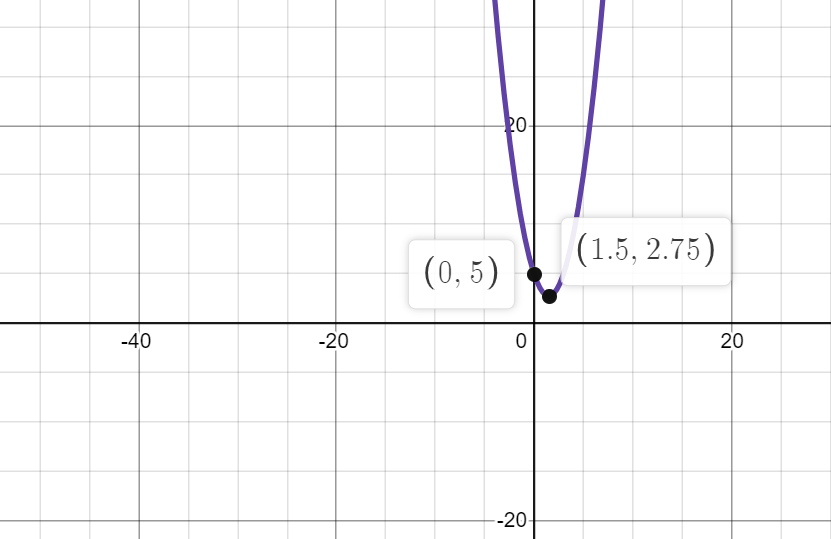### Still have math questions?

Algebra
QuestionConsider the given function, $$m ( x ) = x ^ { 2 } - 3 x + 5 .$$

(a) State whether the graph of the parabola opens upward or downward.

(b) Identify the vertex.

(c) Determine the $$x$$ -intercept(s).

(d) Determine the $$y$$ -intercept(s).

(e) Sketch the function.

(f) Determine the axis of symmetry.

(g) Determine the minimum or maximum value of the function.

(h) Write the domain and range in interval notation.